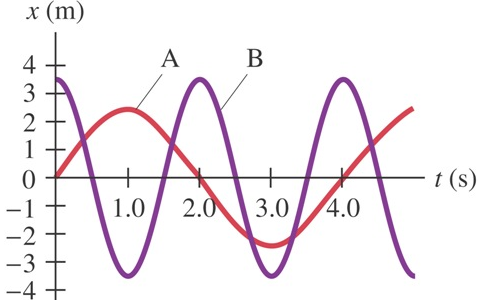# Problem: The figure below shows two examples of SHM (simple harmonic motion), labeled A and B. For each, what is (a) the amplitude, (b) the period, and (c) the frequency and the angular frequency? (d) Write the equations for both A and B in the form of a sine or cosine.

###### FREE Expert Solution

(a)

Amplitude is the maximum displacement from the equilibrium position.

From the graph, amplitude for A:

82% (295 ratings)###### Problem Details

The figure below shows two examples of SHM (simple harmonic motion), labeled A and B. For each, what is

(a) the amplitude,

(b) the period, and

(c) the frequency and the angular frequency?

(d) Write the equations for both A and B in the form of a sine or cosine.